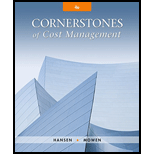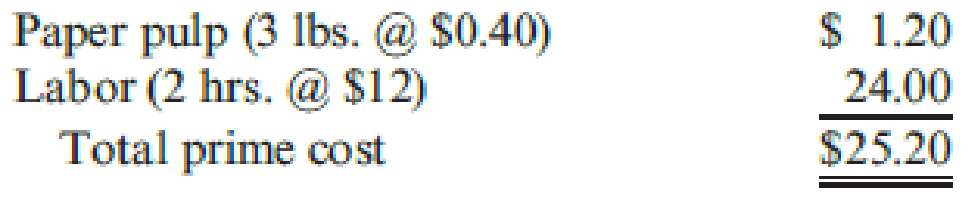# Jameson Company produces paper towels. The company has established the following direct materials and direct labor standards for one case of paper towels: During the first quarter of the year, Jameson produced 45,000 cases of paper towels. The company purchased and used 135,700 pounds of paper pulp at $0.38 per pound. Actual direct labor used was 91,000 hours at$12.10 per hour. Required: 1. Calculate the direct materials price and usage variances. 2. Calculate the direct labor rate and efficiency variances. 3. Prepare the journal entries for the direct materials and direct labor variances. 4. Describe how flexible budgeting variances relate to the direct materials and direct labor variances computed in Requirements 1 and 2.### Cornerstones of Cost Management (C...

4th Edition
Don R. Hansen + 1 other
Publisher: Cengage Learning
ISBN: 9781305970663

#### Solutions

Chapter
Section### Cornerstones of Cost Management (C...

4th Edition
Don R. Hansen + 1 other
Publisher: Cengage Learning
ISBN: 9781305970663
Chapter 9, Problem 20E
Textbook Problem
69 views

## Jameson Company produces paper towels. The company has established the following direct materials and direct labor standards for one case of paper towels:During the first quarter of the year, Jameson produced 45,000 cases of paper towels. The company purchased and used 135,700 pounds of paper pulp at $0.38 per pound. Actual direct labor used was 91,000 hours at$12.10 per hour.Required: 1. Calculate the direct materials price and usage variances. 2. Calculate the direct labor rate and efficiency variances. 3. Prepare the journal entries for the direct materials and direct labor variances. 4. Describe how flexible budgeting variances relate to the direct materials and direct labor variances computed in Requirements 1 and 2.

1.

To determine

Compute the direct materials price variance and the direct materials usage variance.

### Explanation of Solution

Direct material price variance: The variation in between actual price and estimated price paid for materials multiplied by the actual quantity is called material price variance. It is used to determine difference in price paid for material the price that was supposed to be paid for material.

The following formula is used to calculate direct material price variance:

Direct materials price variance=[(ActualPriceStandard Price)×Actual Quantity]

Direct material usage (efficiency) variance: It is a measure that determines the variation in between actual and standard quantity of input multiplied by the standard unit price is called material usage variance.

The following formula is used to calculate direct material usage variance:

Direct materials usage variance=[(ActualQuantityStandard Quantity)×Standard Price]

Compute the direct materials price variance:

Direct materials price variance=[(ActualPriceStandard Price)×Actual Quantity]=[($0.38 per pound$0

2.

To determine

Calculate the direct labor rate variance and labor efficiency variance.

3.

To determine

Prepare journal entries for direct materials and direct labor variances.

4.

To determine

Explain how flexible budgeting variances related to the direct materials and direct labor variances.

### Still sussing out bartleby?

Check out a sample textbook solution.

See a sample solution

#### The Solution to Your Study Problems

Bartleby provides explanations to thousands of textbook problems written by our experts, many with advanced degrees!

Get Started